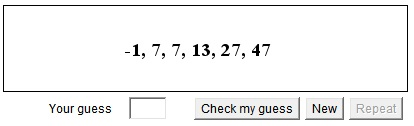# What's next?

The applet below presents you with a sequence of numbers. It's your task to surmise the pattern and guess what would be the next term. Sometimes a pattern may not be unique. This may be very frustrating for which I am sorry. However, this exercise besides being useful and sometimes also enjoyable occupation, affords an opportunity to discuss topics that I consider interesting.

### If you are reading this, your browser is not set to run Java applets. Try IE11 or Safari and declare the site https://www.cut-the-knot.org as trusted in the Java setup.What if applet does not run?## Algorithms used

The applet randomly selects one of the following algorithms. In turn, each of the algorithms has its own random parameters which are mostly coefficients in various formulas.

• Arithmetic series.
a0, a0 + d, a0 + 2d, a0 + 3d, ...

• Geometric series.
a0, a0q, a0q2, a0q3, ...

a0, a1 = a0 + d, a2 = a1·q, a3 = a2 + d, ...

• Alternate addition of two different quantities.
a0, a1 = a0 + da, a2 = a1 + db, ...

• Self-descriptive sequence.
Write a simple number, say, 21. Now scan the number by groups of equal digits at a time. Here we have one "2" and one "1". Thus the next number will be 1211. In this number we have one "1", one "2" and two "1"s. Thus the third number - 111221. The next will be 312211.

• Sum of preceding two.
a0 and a1 are random. a2 = a0 + a1, a3=a1 + a2, ... (If a0 = 0 and a1 = 1,, the rseult is the famous Fibonacci sequence.)

• Three-term sum sequence.
a0, a1 and a2 are random. a3 = a0 + a1 + a2, ...

• Shifted powers.
an = a0 + bqn
Just add a constant to all the members of a geometric series and what a difference!

• Second degree polynomial.
an = an2 + bn + c

It's easy to detect this case. Take differences of successive terms:

 Mn = an + 1 - an = a((n + 1)2 - n2) + b((n + 1) - n) + (c - c) = a(2n + 1) + b

So that the differences prove to be the values of a first order polynomial. Taking "second order" differences we get constants:

 Mn - Mn-1 = a((2n + 1) - (2n - 1)) + (b - b) = 2a

Note: it is very important that you understand that the patterns you looking for in this kind of exercises are not necessarily serve the intended answer. Yes, they are what is expected in official examinations, but rarely in, say, intelligence tests or at job interviews. As an example, what do you think is the next number in the sequence 7, 14, 21, 28?### What is the next number in the sequence 7, 14, 21, 28?

No doubt you thought that it should be 35, right? 35 comes naturally the moment you detect the "arithmetic progression" pattern. However, there are many ways to look at the given sequence. Assume we list the dates (omitting the month) of consecutive Mondays, say. Then, if the given four are the March's Mondays then the first April Monday will be April 4, so that the sequence would be 7, 14, 21, 28, 4. If the sequence is that of April's Mondays, then the continuation is different: 7, 14, 21, 28, 5; for, the next Monday is May 5. It is more confusing if the listed Mondays happened to be in February. For the leap year, the next term would be 6; for the regular year it is 7.

You see, you can't always assume a definite answer.

Another curiousity comes from an article by Roger Penrose: Find the missing number in the sequence

7, 9, 12, ?, 24, 36, 56, 90